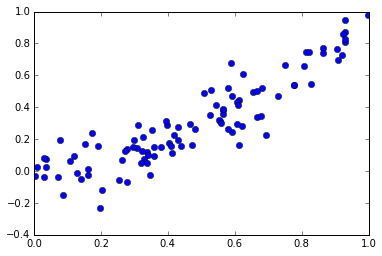Hopefully this includes a notebook...

# A dumb notebook¶

In :
from __future__ import print_function

import numpy as np
import matplotlib.pyplot as plt

%matplotlib inline


## Let's make a plot¶

Of some random data.

In :
# make some points...
x = np.random.random(100)

# and a function of them
y = x**2

y += np.random.randn(100)/10

In :
plt.plot(x,y, 'o')

Out:
[<matplotlib.lines.Line2D at 0x1088021d0>]In [ ]: